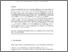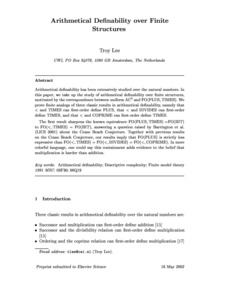# PP-2002-04: Arithmetical Definability over Finite Structures

PP-2002-04: Lee, Troy (2002) Arithmetical Definability over Finite Structures. [Report]Preview Text (Full Text (PDF)) PP-2002-04.text.pdf Download (233kB) | PreviewText (Full Text (PS)) PP-2002-04.text.ps.gz Download (82kB)Text (Abstract) PP-2002-04.abstract.txt Download (1kB)

## Abstract

Arithmetical definability has been extensively studied over the
natural numbers. In this paper, we take up the study of arithmetical
definability over finite structures, motivated by the correspondence
between uniform \$\AC^0\$ and \$\FO(\PLUS,\TIMES)\$. We prove finite
analogs of three classic results in arithmetical definability, namely
that \$<\$ and TIMES can first-order define PLUS, that \$<\$ and DIVIDES
can first-order define TIMES, and that \$<\$ and COPRIME can first-order
define TIMES.

The first result sharpens the known equivalence
\${\FO(\PLUS,\TIMES)=}{\FO(\BIT)}\$ to \$\FO(<,\TIMES)=\FO(\BIT)\$,
answering a question raised by Barrington et al. (LICS 2001) about the
Crane Beach Conjecture. Together with previous results on the Crane
Beach Conjecture, our results imply that \$\FO(\PLUS)\$ is strictly less
expressive than \$\FO(<,\TIMES)=\FO(<,\DIVIDES)=\FO(<,\COPRIME)\$. In
more colorful language, one could say this containment adds evidence
to the belief that multiplication is harder than addition.

Item Type: Report PP-2002-04 Prepublication (PP) Series 2002 Arithmetical Definability; Descriptive Complexity; Finite Model Theory 12 Oct 2016 14:36 12 Oct 2016 14:36 https://eprints.illc.uva.nl/id/eprint/68View Item